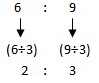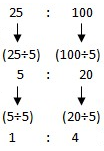### Sample Problem

Find the simplest form of the following ratios.

(a) 6:9=

(b) 25:100=

#### Solution

Always express ratios in term of whole numbers in their lowest terms. Thus,

Divide each part of the given ratios by common factors until they cannot be divided by any factors.

(a)2 and 3 cannot be divided further by a common factor.

(b)1 and 4 cannot be divided further by a common factor.

In ratios such as 2:3 and 1:4, all numbers cannot be divided by any common factor. Such ratios are in their simplest form.